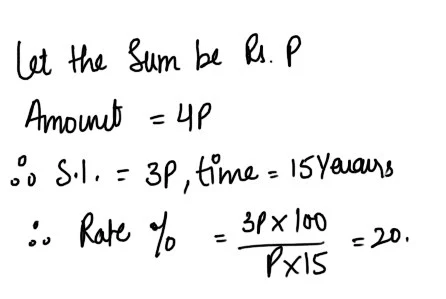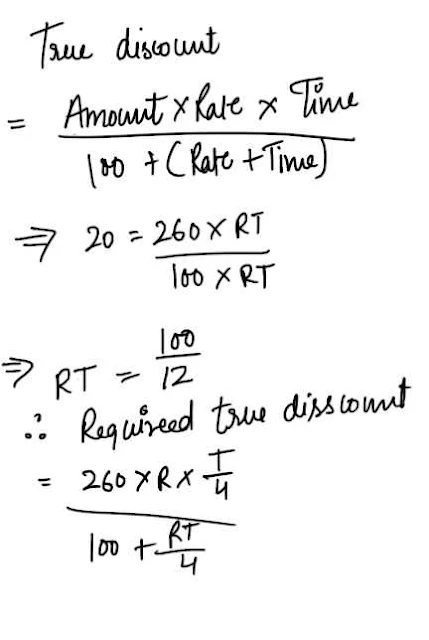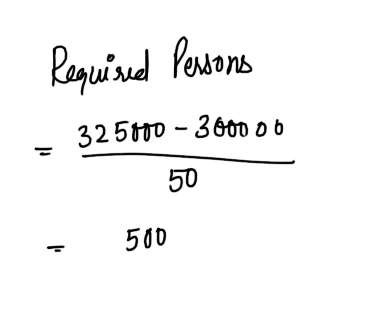New Student User - Use Code HELLO

# Mixed Aptitude Quiz - 4 (For SBI Clerical 2016)

#### Ques 1.

The average age of 40 students of a class is 15 years. When 10 new students are admitted, then the average in increased by 0.2 year.The average age of the new students is
(a) 15.2 years

(b) 16 years
(c) 16.2 years
(d) 16. 4 years
Ans 1.#### Ques 2.

The rate at which a sum becomes four times of itself in 15 years at simple interest will be
(a) 25 %
(b) 20%
(c) 17 1/2%
(d) 15%
Ans 2.#### Ques 3.

How many words can be formed from the letters of the word SIGNATURE so that the vowels always come together?
(a) 2880
(b) 1400
(c) 17280
(d) 3600
Ans 3. The word SIGNATURE  consists of nine letters  comprising four vowels (A,E, I nd U) and five consonants (G,N,R,T and S). when the four vowels are considered as one letter, we have six letters which can be arranged in 6P6 ways,i.e. 6! ways. Note that the four vowels can be arranged in 4! ways.
Hence, required number of words = 6! x 4!
= 720 x 24 = 17280.

#### Ques 4.

Rs.20 is the true discount on Rs. 260 due after a certain time. What will be the true discount on the same sum due after a quarter of the former time , the rate of interest being the same ?
(a) Rs 5 15/49
(b) Rs 4 15/49
(c) Rs 5 16/49
(d) Rs 4 16/49
Ans 4.#### Ques 5.

A can finish a piece of work in 5 Days.B can do it in 10 days. They work together for two days an then A goes away. In how many days will B finish the work ?
(a) 4 days
(b) 5 days
(c) 6 days
(d) 8 days
Ans 5.#### Ques 6.

What is the sum of all odd numbers upto 100?
(a) 2650
(b) 2600
(c) 2500
(d) 2560
Ans 6. The odd numbers  are :
1, 3, 5, 7.....99
The sequence is an A.P. with a = 1 and d =
2. The number of terms is 50.
. . Required sum = 50/2 [ 1+ 99]
= 2500.

#### Ques 7.

If k + 1 is an odd integer, which of the following must also be an off integer?
(a) 2 (k +1)
(b) ( k+1)k
(c) (k+1) (k+2)
(d)_ (k+1)(k-1)
Ans 7. k is an even integer
. . k - 1 is odd
=> (k+1) ( k-1) is odd.

#### Ques 8.

5 kg of sugar costing Rs. 8 per kg and 3 kg of sugar costing Ts. 6 per kg are mixed .What will be the average cost of the mixture?
(a) Rs 7.25 per kg
(b) Rs 7.50 per kg
(c) Rs 7 per kg
(d) None of these
Ans 8.#### Ques 9.

N number of persons decide to raise Rs 3 lakhs by equal contribution from each.If they contributed Rs.50 each extra, the contribution would be Rs 3.25 lakhs .How many persons are there?
(a) 600
(b) 400
(c) 350
(d) None of these
Ans 9.#### Ques 10.

Milk contains 5%water . What quantity of pure milk should be added to 10 litres of milk to reduce this to 2%?
(a) % litres
(b) 10 litres
(c) 15 litres
(d) 7 litres
Ans 10.